#Function Repository Resource:

# MultivariateMedianDeviation

Find the median Euclidean distance from the median of the elements of multivariate data

Contributed by: Wolfram Research
 ResourceFunction["MultivariateMedianDeviation"][matrix] gives the median Euclidean distance from the median of the elements in matrix.

## Details and Options

ResourceFunction["MultivariateMedianDeviation"] is a univariate measure of median deviation for multivariate data.
The multivariate median deviation is given by the median of the list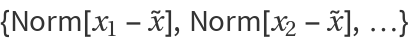, where matrix={x1,x2,,xn} and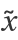is the median of matrix.
The following option can be given:
 "MedianMethod" Median the median to use
Valid settings for "MedianMethod" are "Median", "SimplexMedian", "ConvexHullMedian" and "SpatialMedian".

## Examples

### Basic Examples

Multivariate median deviation for bivariate data:

 In:=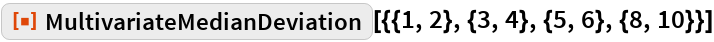Out=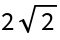### Options

#### MedianMethod

Multivariate median deviation using a simplex median:

 In:=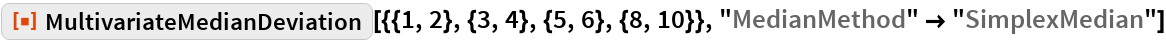Out=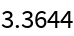## Requirements

Wolfram Language 11.3 (March 2018) or above